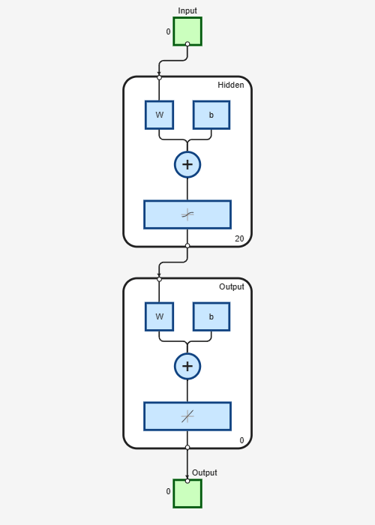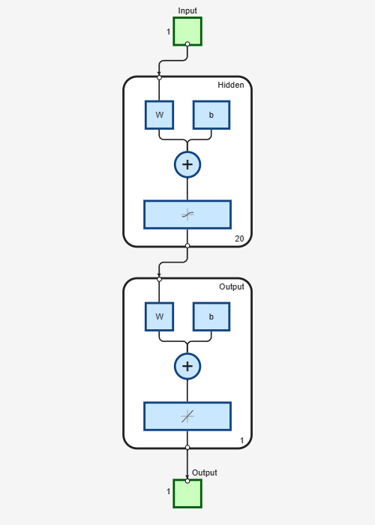# configure

Configure network inputs and outputs to best match input and target data

## Syntax

``net = configure(net,x,t)``
``net = configure(net,x)``
``net = configure(net,'inputs',x,i)``
``net = configure(net,'outputs',t,i)``

## Description

example

````net = configure(net,x,t)` takes input data `x` and target data `t`, and configures the network’s inputs and outputs to match. Configuration is the process of setting network input and output sizes and ranges, input preprocessing settings and output postprocessing settings, and weight initialization settings to match input and target data.Configuration must happen before a network’s weights and biases can be initialized. Unconfigured networks are automatically configured and initialized the first time `train` is called. Alternately, a network can be configured manually either by calling this function or by setting a network’s input and output sizes, ranges, processing settings, and initialization settings properties manually.```
````net = configure(net,x)` configures only inputs.```
````net = configure(net,'inputs',x,i)` configures the inputs specified with the index vector `i`. If `i` is not specified all inputs are configured.```
````net = configure(net,'outputs',t,i)` configures the outputs specified with the index vector `i`. If `i` is not specified all targets are configured.```

## Examples

collapse all

This example shows how to manually configure a network for a simple fitting problem instead of using the train function.

```[x,t] = simplefit_dataset; net = feedforwardnet(20); view(net)``````net = configure(net,x,t); view(net)```## Input Arguments

collapse all

Input network, specified as a network object. To create a network object, use for example, `feedforwardnet` or `narxnet`.

Network inputs, specified as a matrix.

Network targets, specified as a matrix.

Indexes of the inputs or outputs you want to configure, specified as a vector.

## Output Arguments

collapse all

Configured network, returned as a network object.

## Version History

Introduced in R2010b